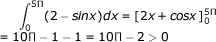# Engineering Maths - Calculus

> > > > > > > > Calculus

## Calculus GATE  Syllabus

Calculus: Limits, continuity and differentiability. Maxima and minima. Mean value theorem. Integration

• A

2x2• B

√x• C

0• D

1• Option : A
• Explanation :• A

a maxima at x= 1 and a minima at x = 3• B

a maxima at x = 3 and a minima at x = 1• C

no maxima, but a minima at x = 1• D

a maxima at x = 1, but no minima• Option : A
• Explanation :

f '(x) = 3(x2-4x+3)    f ''(x)=6(x)-12 at  x=1,  f "(1) at  x=3, f ''(3) = +ve

• A

>0• B

2• C

0 - 1 + 100 - 10 + 1• D

undefined• Option : A
• Explanation :• A

[-3, 3]• B

[-2, 2]• C

[2, 3]• D

[-1, 1]• Option : C
• Explanation : Since f(x) = (1/x) is not continuous in [- 3, 3] [- 4, 2] or [- 1, 1], The point of discontinuity is '0'. Only in [2, 3] the function is continuous, and differentiable hence mean value theorem is applicable in [2, 3].

• A

satisfied all the conditions of Rolle's Theorem• B

satisfied all the conditions of Mean Value Theorem• C

does not satisfied the -conditions of Mean Value Theorem• D

None of these• Option : C
• Explanation : Since f(x) = |x| is continuous in [-1, 1] but it is not differentiable at x = 0 ε (-1, 1)

## Description

• These calculus multiple choice questions answers can be used by any Engineering student to gain good credit score.
• Calculus Objective Type questions answers can also be used by FY B. Sc. Students to prepare for their exams.
• B. Sc. Maths and M.Sc. Maths students can also use these Calculus Practice Questions.
• Students who are are pursuing their XI, XII standard can also used these Calculus Practice Questions.

Various search terms used for this section are

Related Quiz.
Calculus